Published: 31 December 2017

# An analytical modeling for high-velocity impacts on woven Kevlar composite laminates

Chen Fangyu1
Zhou Li2
Tang Yihao3
Zhou Xiaoming4
1, 2State Key Laboratory of Mechanics and Control of Mechanical Structures, Nanjing University of Aeronautics and Astronautics, Nanjing, China
3, 4China Helicopter Research and Development Institute, Jingdezhen, China
Corresponding Author:
Zhou Li
Views 161

#### Abstract

In this paper, an analytical model, which based on energy balance, is built to study the process of high velocity impacts on woven Kevlar composite laminates by a cylindrical projectile. Four different mechanisms, such as laminate crushing, linear momentum transfer and tensile fiber failure, and shear plugging, is absorbed by the laminate while impacting. Then, simplification of the model is done to obtain the residual velocity and ballistic limit. The analytical results are validated with the results of experiment, and the perturbation analysis is done to analyze the reason of error.

## 1. Introduction

Resistance to ballistic impacts is an important requirement in many industrial applications, such as car, space shuttle and aeronautical industry. Woven fabrics are well-known to be good choice to resist ballistic impacts [1-3]. In the past, several researches have been focused on the impact on composite laminates. Elasticity method [4-7] was widely used to predict the behavior of impact. Three phases, which namely indentation, perforation and exit, are divided in the impact event by Zhu et al. . Sun et al.  applied different criteria to obtain damage initiation, progression and plug formation. Wen et al. [6, 7] applied projectiles with different shapes to investigate FRP laminates’ perforation and penetration. Another method to predict the behavior of impact was the energy method. The projectile’s residual velocity and ballistic limit are calculated by energy balance equation. Naik et al.  and Xuan Haijun et al.  study the perforation of the composite plates with all the damage mechanisms and verified the analytical results with experimental results. Impact test is done by Ganesh Babu et al.  using different shape projectiles on unidirectional glass/epoxy composite plates. Sikarwar et al. [11, 12] and Wang Yuanbo et al. [13, 14] studied the analytical and experimental results of the residual velocity and ballistic limit of glass/epoxy and Kevlar/epoxy composite laminates respectively, which are subjected to high velocity impact. Yahaya et al.  observed a linear increasing tendency of energy absorption against impact velocity for Kevlar-Kenaf hybrid woven composites. Gu Xingjin et al.  and Michele et al.  calculate energy absorbed in different mechanisms with flat-end cylindrical projectiles. Chen et al.  studied simulation and experimental results of damage and energy absorption behavior for woven composites under ballistic impact. The researches mentioned above spend a lot of attention to high precision of the solutions of different equations, so they are high cost. To make a quick assessment at early stages of the design process, Lopez-Puente et al. neglect elastic deformation contribution in impact event  and then proposes a low-cost model and obtains closed-form solution to predict the ball projectile’s residual velocity after impacting on thin CFRPs woven laminated plates , but the influence of shear plugging [21-23] is not considered in their research.

In this paper, an analytical model is proposed to build the energy balance equation and predict the residual velocity of a cylindrical projectile after impacting at woven Kevlar composites. Simplification of the model is done to obtain the residual velocity and ballistic limit. Also, three dimensionless variables are built to simplify the process of analysis perturbation, which caused by tensile fiber failure. Analysis is done to explain the error between the results of calculation and experiment . The model is low-cost, and its predictive accuracy is improved. The method of simplification could be useful in preliminary assessment of engineering.

## 2. Analytical model

The analytical model is built by terms of energy balance. In Lopez-Puente et al.’  opinion, the kinetic energy of the projectile ${E}_{k}$ is absorbed by the laminate through three different processes. ${E}_{c}$ is the energy of crushing, which leads to laminate breakage. ${E}_{m}$ is the energy of linear momentum, transferred from the projectile to the detached part of laminate. ${E}_{f}$ is the energy of tensile fiber failure breakage. Elastic deformation energy is not considered by them in this analytical model. Naik et al. [19, 20]and Brenda et al.  consider that ${E}_{sp}$, the energy absorbed by shear plugging, is also an important process. So, the basic balance equation can be presented in the following because of the influence of the shear plugging:

1
$-d{E}_{k}=d{E}_{c}+{E}_{m}+d{E}_{f}+d{E}_{sp}.$

Following each energetic term of Eq. (1) is presented.

The kinetic energy of the projectile is expressed as:

2
$d{E}_{k}\left(x\right)=\frac{1}{2}{m}_{p}d\left(v{\left(x\right)}^{2}\right),$

where $x$ is the distance between the position of the projectile and the impact surface of the composite laminate, ${m}_{p}$ is the mass of projectile, and $v$ is the velocity varies with the position.

The energy of laminate crushing is expressed as:

3
$d{E}_{c}\left(x\right)={\sigma }_{c}A\left(x\right)dx,$

where $A\left(x\right)$ is the frontal projectile area that contacts the non-crushed laminate and ${\sigma }_{c}$ is the out-of-plane compressive strength of the laminate. Here, $A\left(x\right)=\pi {r}^{2}$, where $r$ denotes the radius of the projectile cross-section.

The energy of linear momentum transfer is expressed as:

4
$d{E}_{m}\left(x\right)=\frac{1}{2}\left(A\left(x\right)dx\rho \right){v}^{2}\left(x\right),$

where $\rho$ is the density of laminate.

The energy of tensile fiber failure is expressed as:

5
$d{E}_{f}\left(x\right)={\omega }_{f}dV,$

where ${\omega }_{f}$ is the specific energy and $dV$ is the affected material volume at any $dx$. The specific energy is estimated as:

6
${\omega }_{f}=2\left(\frac{1}{2}{X}_{t}{\epsilon }_{f}\right),$

where ${X}_{t}$ is the tensile strength in fiber direction and ${\epsilon }_{f}$ is the ultimate strain. Experimental result [9, 20] shows that the transverse section of laminate is a truncated pyramid, with a semi-angle $\alpha$ and $\beta$, and its diagonals aligned in the fiber directions. The upper base semi-diagonal is $r$ and the lower base semi-diagonal is $L=r+\left(h-{x}_{0}\right)\mathrm{t}\mathrm{a}\mathrm{n}\alpha$ with ${x}_{0}$ the position of the projectile at the time ${x}_{0}$, as Fig. 1 shown. The affected material volume $dV$ is given by:

7
$dV=2l×{l}_{w}dx=2dx{\left(r+\left(h-{x}_{0}\right)\mathrm{t}\mathrm{a}\mathrm{n}\alpha \right)}^{2}\mathrm{t}\mathrm{a}\mathrm{n}\beta ,$

and the energy of tensile fiber failure is given by:

8
$d{E}_{f}\left(x\right)=2{X}_{t}{\epsilon }_{f}{\left(r+\left(h-{x}_{0}\right)\mathrm{t}\mathrm{a}\mathrm{n}\alpha \right)}^{2}\mathrm{t}\mathrm{a}\mathrm{n}\beta dx.$

The value of ${x}_{0}$ can be obtained from following equation:

9
${\int }_{0}^{{x}_{0}}\frac{dx}{v\left(x\right)}=\frac{2h}{\sqrt{{E}_{3}/\rho }},$

where $h$ is the thickness of laminate and ${E}_{3}$ is the elastic modulus through-thickness.

Fig. 1Woven composite with cylindrical projectile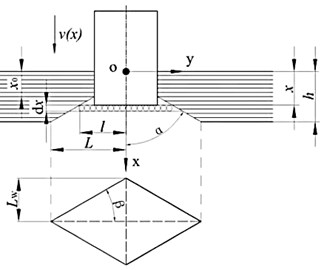Tensile fiber failure happens while ${x}_{0}, so a function ${C}_{r}\left(x\right)$ is defined as follow to multiplies the last equation:

10
${C}_{r}\left(x\right)=\left\{\begin{array}{l}0,0

The energy absorbed of shear plugging is expressed as:

11
$d{E}_{sp}\left(x\right)={S}_{sp}S\left(x\right)xdx,$

where ${S}_{sp}$ is shear plugging strength and $S\left(x\right)$ is the cross-section perimeter of the projectile. Here, $S\left(x\right)=2\pi r$.

While $x\ge {x}_{0}$, the crushing energy and shear plugging energy have no influence. So, a function ${C}_{s}\left(x\right)$ is defined as follow to multiply the Eq. (3) and Eq. (4):

12
${C}_{s}\left(x\right)=\left\{\begin{array}{l}1,0

## 3. Model simplification

As analysis above, the basic balance equation can be written as:

13
$-\frac{1}{2}{m}_{p}d\left(v{\left(x\right)}^{2}\right)={\sigma }_{c}A\left(x\right){C}_{s}\left(x\right)dx+\frac{1}{2}\left(A\left(x\right)dx\rho \right){v}^{2}\left(x\right)$
$+2{X}_{t}{\epsilon }_{f}{\left(r+\left(h-{x}_{0}\right)\mathrm{t}\mathrm{a}\mathrm{n}\alpha \right)}^{2}\mathrm{t}\mathrm{a}\mathrm{n}\beta {C}_{r}\left(x\right)dx+{S}_{sp}S\left(x\right)x{C}_{s}\left(x\right)dx.$

To solve the equation, some simplifications are made and the initial condition is imported:

14
$\left\{\begin{array}{l}-\frac{1}{2}{m}_{p}\frac{{d}^{2}v}{dx}=\frac{1}{2}\left(A\left(x\right)\rho \right){v}^{2}+{S}_{sp}S\left(x\right)x{C}_{s}\left(x\right)+{\sigma }_{c}A\left(x\right){C}_{s}\left(x\right)\\ +2{X}_{t}{\epsilon }_{f}{\left(r+\left(h-{x}_{0}\right)\mathrm{t}\mathrm{a}\mathrm{n}\alpha \right)}^{2}\mathrm{t}\mathrm{a}\mathrm{n}\beta {C}_{r}\left(x\right),\\ v\left(0\right)={v}_{0}.\end{array}\right\$

Here the velocity and the displacement are converted to dimensionless variables:

15
${v}^{*}=\frac{v}{{v}_{0}},{x}^{*}=\frac{x}{h}.$

By defining a variable ${y}^{*}={v}^{{*}^{2}}$, Eq. (14) leads to:

16
$\left\{\begin{array}{l}-\frac{d{y}^{*}}{d{x}^{*}}=\frac{\pi {r}^{2}h\rho }{{m}_{p}}{y}^{*}+\frac{4{S}_{sp}\pi r{h}^{2}}{{m}_{p}{v}_{0}^{2}}{C}_{s}\left(h{x}^{*}\right){x}^{*}+\frac{2\pi {r}^{2}h{\sigma }_{c}}{{m}_{p}{v}_{0}^{2}}{C}_{s}\left(h{x}^{*}\right)\\ +\frac{4{X}_{t}{\epsilon }_{f}{r}^{2}h}{{m}_{p}{{v}_{0}}^{2}}{\left(1+\left(\frac{h}{r}{x}^{*}-\frac{{x}_{0}}{r}\right)\mathrm{t}\mathrm{a}\mathrm{n}\alpha \right)}^{2}\mathrm{t}\mathrm{a}\mathrm{n}\beta {C}_{r}\left(h{x}^{*}\right),\\ {y}^{*}\left(0\right)=1.\end{array}\right\$

To simplify the process of finding solution, the relative importance of every energy absorption mechanisms are estimated firstly. Then, inconsequential factors can be found and ignored.

The characteristic values of different variables involved are showed in Table 1.

Some variables are defined as a function of the impact velocity to estimate the relative importance of the terms that appear in the equation:

17
$\left\{\begin{array}{l}{R}_{m}=\frac{\pi {r}^{2}h\rho }{{m}_{p}}-1{0}^{-2},\\ {R}_{sp}=\frac{4{S}_{sp}\pi r{h}^{2}}{{m}_{p}{v}_{0}^{2}}-\frac{1{0}^{3}}{{v}_{0}^{2}},\\ {R}_{c}=\frac{2\pi {r}^{2}h{\sigma }_{c}}{{m}_{p}{v}_{0}^{2}}-\frac{1{0}^{3}}{{v}_{0}^{2}},\\ {R}_{f}=\frac{4{X}_{t}{\epsilon }_{f}{r}^{2}h}{{m}_{p}{v}_{0}^{2}}-\frac{10}{{v}_{0}^{2}}.\end{array}\right\$

Obviously, the value of ${R}_{f}$ is very small. Within the velocity range considered, ${R}_{f}$ is always lower than the others. The energy of tensile fiber failure is ignored to simplify the model as following:

18
$\left\{\begin{array}{l}-\frac{d{y}^{*}}{d{x}^{*}}=\frac{\pi {r}^{2}h\rho }{{m}_{p}}{y}^{*}+\frac{4{S}_{sp}\pi r{h}^{2}}{{m}_{p}{v}_{0}^{2}}{C}_{s}\left(h{x}^{*}\right){x}^{*}+\frac{2\pi {r}^{2}h{\sigma }_{c}}{{m}_{p}{v}_{0}^{2}}{C}_{s}\left(h{x}^{*}\right),\\ {y}^{*}\left(0\right)=1.\end{array}\right\$

The equation can be numerically solved to find the solution:

19
${y}^{*}=\left\{\begin{array}{l}-\frac{4{S}_{sp}h}{{v}_{0}^{2}r\rho }{x}^{*}+\frac{4{S}_{sp}{m}_{p}}{{v}_{0}^{2}\pi {r}^{3}{\rho }^{2}}-\frac{2{\sigma }_{c}}{{v}_{0}^{2}\rho }+{e}^{-\frac{\pi {r}^{2}h\rho }{{m}_{p}}{x}^{*}}\left(-\frac{4{S}_{sp}{m}_{p}}{{v}_{0}^{2}\pi {r}^{3}{\rho }^{2}}+\frac{2{\sigma }_{c}}{{v}_{0}^{2}\rho }+1\right),0<{x}^{*}<{x}_{0}^{*},\\ -\frac{4{S}_{sp}h}{{v}_{0}^{2}r\rho }{x}_{0}^{*}+\frac{4{S}_{sp}{m}_{p}}{{v}_{0}^{2}\pi {r}^{3}{\rho }^{2}}-\frac{2{\sigma }_{c}}{{v}_{0}^{2}\rho }+{e}^{-\frac{\pi {r}^{2}h\rho }{{m}_{p}}{x}_{0}^{*}}\left(-\frac{4{S}_{sp}{m}_{p}}{{v}_{0}^{2}\pi {r}^{3}{\rho }^{2}}+\frac{2{\sigma }_{c}}{{v}_{0}^{2}\rho }+1\right),{x}^{*}\ge {x}_{0}^{*}.\end{array}\right\$

${x}_{0}^{*}$ can be obtained from following equation:

20
${\int }_{0}^{{x}_{0}^{*}}\frac{{v}_{0}}{\sqrt{{y}^{*}\left({x}^{*}\right)}}d{x}^{*}=\frac{2h}{\sqrt{{E}_{3}/\rho }}.$

The expression for residual velocity is obtained, which is given by:

21
${v}_{r}=\underset{{x}^{*}\to \infty }{\mathrm{l}\mathrm{i}\mathrm{m}}v\left({x}^{*}\right)=\sqrt{-\frac{4{S}_{sp}h}{r\rho }{x}_{0}^{*}+\frac{4{S}_{sp}{m}_{p}}{\pi {r}^{3}{\rho }^{2}}-\frac{2{\sigma }_{c}}{\rho }+{e}^{-\frac{\pi {r}^{2}h\rho }{{m}_{p}}{x}_{0}^{*}}\left(-\frac{4{S}_{sp}{m}_{p}}{\pi {r}^{3}{\rho }^{2}}+\frac{2{\sigma }_{c}}{\rho }+{v}_{0}^{2}\right)}.$

For ballistic limit ${v}_{50}$, ${v}_{r}=$ 0, so ballistic limit ${v}_{50}$ can be expressed by:

22
${v}_{50}=\sqrt{\frac{\frac{4{S}_{sp}h}{r\rho }{x}_{0}^{*}-\frac{4{S}_{sp}{m}_{p}}{\pi {r}^{3}{\rho }^{2}}+\frac{2{\sigma }_{c}}{\rho }-{e}^{-\frac{\pi {r}^{2}h\rho }{{m}_{p}}{x}_{0}^{*}}\left(-\frac{4{S}_{sp}{m}_{p}}{\pi {r}^{3}{\rho }^{2}}+\frac{2{\sigma }_{c}}{\rho }\right)}{{e}^{-\frac{\pi {r}^{2}h\rho }{{m}_{p}}{x}_{0}^{*}}}}.$

Table 1Characteristic values of different variables

 Variable Characteristic values $h$ [m] 10-3 $r$ [m] 10-3 ${m}_{p}$ [kg] 10-3 $\rho$ [kg/m3] 103 ${X}_{t}$ [N/m2] 109 ${\sigma }_{c}$ [N/m2] 108 ${S}_{sp}$ [N/m2] 108 ${\epsilon }_{f}$ 10-2

## 4. Model validation and discussion

To validate the analytical model above, analytical results are calculated to compare with experimental result . Here cylindrical projectiles with a diameter of 7.62 mm and a mass of 5.2 g are used to impact 0°/90° layup Kevlar laminates with thickness of 5 mm and 10 mm respectively. The mechanical properties of Kevlar composite are shown in Table 2.

The projectile residual velocities obtained from different laminate thickness values and projectile initial velocities are shown as Fig. 2. It is clear that the differences between results of calculation and experiment reduce as the initial velocity increases for laminates. For laminate with thickness of 5 mm, the error is about 13 % while the initial velocity below 400 m/s, and the error is less than 9 % while the initial velocity over 400 m/s. It shows that the calculated result is closer to experimental result for laminates with thickness of 10 mm than that of 5 mm. Also, good result was found in the residual velocity estimated by the mentioned method , but its error is larger than that found by the proposed model.

The ballistic limit obtained for different laminate thickness values are shown as Fig. 3.

It is clear that the difference between calculated result and experimental result is larger while the laminates with thickness of 5 mm than which of 10 mm. The ballistic limit of calculated result  is lower than that of proposed model.

Table 2Mechanical properties of Kevlar composites

 Mechanical property Value $\rho$ [kg/m3] 1440 Modulus in $x$ direction ${E}_{x}$ [GPa] 21 Modulus in $y$ direction ${E}_{y}$ [GPa] 21 Modulus in $z$ direction ${E}_{z}$ [GPa] 4.6 Shear modulus in $xy$ direction ${G}_{xy}$ [GPa] 5.2 ${X}_{t}$ [MPa] 800 ${S}_{sp}$ [MPa] 183 ${\sigma }_{c}$ [MPa] 80 ${\epsilon }_{f}$ 0.02

Fig. 2Comparison of residual velocities obtained from analysis and experiment for different projectile initial velocities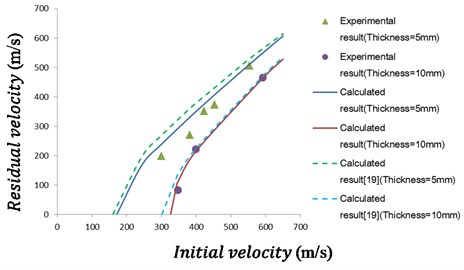Fig. 3Comparison of ballistic limit obtained from analysis and experiment for different laminate thickness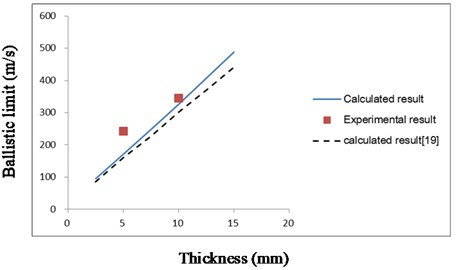The key of explaining the error is analyzing the perturbation caused by tensile fiber failure. It is also important to analyze the influence of the energy absorbed by other three different mechanisms. To simplify the process of analysis and estimate the influence of every mechanisms quickly, three dimensionless variables ${R}_{sp}/{R}_{f}$, ${R}_{m}/{R}_{f}$ and ${R}_{c}/{R}_{f}$ are built.

Fig. 4 shows that the perturbation caused by tensile fiber failure descends as soon as the initial velocity increases. Shear plugging is always the main absorbing energy mechanism (within the velocity range considered). At initial velocity below 370 m/s, the crushing is more important than the momentum transfer. At initial velocity below 100 m/s, the momentum transfer is almost without influence. As the impact velocity increases, the ratio of ${R}_{m}/{R}_{f}$ rises quickly. The reason is that the ratio is proportional to square of the projectile velocity. It means that the influence of shear plugging descends as the initial velocity increases, so the solutions between the proposed model and method  become closer in Fig. 2.

Fig. 5 shows that the perturbation caused by tensile fiber failure descends as soon as the thickness of laminate increases. Shear plugging is the main absorbing energy mechanism while thickness over 1mm. Crushing is always more important than momentum transfer. As the impact velocity increases, the ratio of ${R}_{sp}/{R}_{f}$ rises quickly. The reason is that the ratio is proportional to the thickness of laminate. It means that the influence of shear plugging rises as the initial velocity increases, so the solutions between the proposed model and method  become larger in Fig. 3.

Fig. 4Ratio of different factor for different projectile initial velocities (thickness = 5 mm)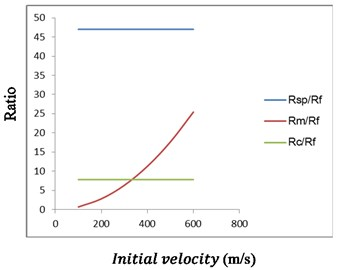Fig. 5Ratio of different factor for different laminate thickness (v0= 200 m/s)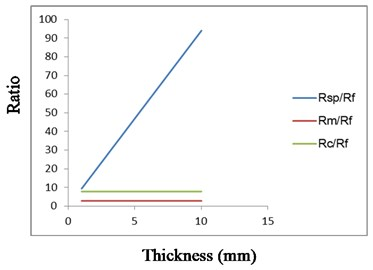## 5. Conclusions

An analytical model to predict residual velocity and ballistic limit after high velocity impact on woven Kevlar laminates is proposed. Four different energy absorption mechanisms for the laminate are considered in the model. Then, the model is simplified to obtain the residual velocity and ballistic limit. The analytical result is calculated to compare with that of experiment. The comparison shows a good correlation between the results obtained both numerically and experimental. The perturbation caused by tensile fiber failure descends as soon as the initial velocity or the thickness of laminate increases. The importance of energy absorbing by shear plugging, crushing and the momentum transfer will change by changing of initial velocity of projectile and thickness of laminate. The method of simplification could be useful in preliminary assessment of engineering.

#### Cited by

Numerical simulation of armor materials and optimization using gray relational analysis